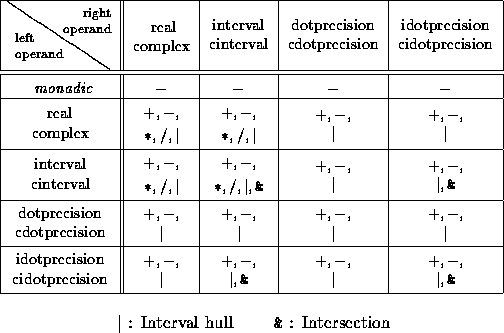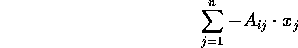Evaluation of Expressions with High AccuracyNext: Dynamic Multiple-Precision Arithmetic Up: C-XSC Previous: Subarrays of Vectors

# Evaluation of Expressions with High AccuracyTable 4: Predefined Dotprecision Operators

When evaluating arithmetic expressions, accuracy plays a decisive role in many numerical algorithms. Even if all arithmetic operators and standard functions are of maximum accuracy, expressions composed of several operators and functions do not necessarily deliver results with maximum accuracy (see ). Therefore, methods have been developed for evaluating numerical expressions with high and mathematically guaranteed accuracy.

A special kind of such expressions are called dot product expressions, which are defined as sums of simple expressions. A simple expression is either a variable, a constant, or a single product of two such objects. The variables may be of scalar, vector, or matrix type. Only the mathematically relevant operations are permitted for addition and multiplication. The result of such an expression is either a scalar, a vector, or a matrix. In numerical analysis, dot product expressions are of decisive importance. For example, methods for defect correction or iterative refinement for linear or nonlinear problems are based on dot product expressions. An evaluation of these expressions with maximum accuracy avoids cancellation. To obtain an evaluation with 1 ulp accuracy, C-XSC provides the dotprecision data types

dotprecision, cdotprecision, idotprecision, and cidotprecision.

Intermediate results of a dot product expression can be computed and stored in a dotprecision variable without any rounding error. The following example computes an optimal inclusion of the defect b - Ax of a linear system Ax = b:

```ivector defect(rvector b, rmatrix A, rvector x)
{
idotprecision accu;
ivector incl(Lb(x),Ub(x));

for (int i=Lb(x); i<=Ub(x); i++) {
accu = b[i];
accumulate(accu, -A[i], x);
incl[i] = rnd(accu);
}
return incl;
}```

In the example above, the function accumulate() computes the sum:and adds the result to the accumulator `accu` without rounding error. The idotprecision variable `accu` is initially assigned `b[i]`. Finally, the accumulator is rounded to the optimal standard interval `incl[i]`. Thus, the bounds of `incl[i]` will either be the same or two adjacent floating-point numbers.

For all dotprecision data types, a reduced set of predefined operators is available to compute results without any error. The overloaded dot product routine accumulate() and the rounding function rnd() are available for all reasonable type combinations.Next: Dynamic Multiple-Precision Arithmetic Up: C-XSC Previous: Subarrays of Vectors

Andreas Wiethoff
Wed Mar 29 13:50:24 MET DST 1995